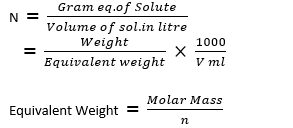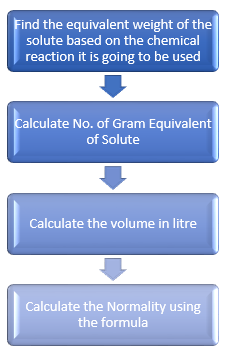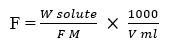# Normality Definition , Formula , Formality Formula, Solved Examples/Problems

Table of Content

## Normality Definition and Formula

We have studied about of the measure of concentration like Molarity, Mole Fraction,Molality.We are going to see another measure of concentration called Normality. Normality is defined as the number of gram equivalent present in per litre solution. It is used for acid or base solutionwhere n =  number of H+ in Acid  , OH- in base and for salt,charge present in ionic forms
Equivalent Weight is new thing in the formula. Lets check how it is defined

Equivalent Weight Definition and Gram Equivalent Weight
Equivalent Weight is defined the as ratio of Molar Mass of substance to the valence of the substance.Valence is also denoted as equivalence factor. The valence is the number of H+ in Acid  , OH- in base and for salt,charge present in ionic forms. For reduction/oxidation (redox) reactions it is number of electrons that an oxidizing or reducing agent can accept or donate are counted as valence or equivalence factor. When the Equivalent Weight is expressed in gram using molar mass in gram, it is called Gram equivalent weight.
Few more important things about Normaility
• Normality unit is N or eq/L .It depends on the chemical reaction being studied and depends on temperature too. So ,it is meaning with respect to the chemical reaction
• It is the reactive capacity of the solution and it is called equivalent concentration
• This is used in acid-base reactions and redox reactions

## Difference Between Normality and Molarity

 Molarity Normality number of moles of solute per liter of solution. number of gram equivalent of solute per liter of solution. Molarity is a measurement of the Moles in the total volume of the solution, Normality  is a measurement of the gram equivalent in relationship to the total volume of the solution. Unit is Moles L-1 Unit is eq L-1

## Relation between Normality and Molarity

Here is Normality formula in terms of molarity
$\text{Normality} = n \times \text{Molarity}$
where n =  number of H+ in Acid  , OH- in base and for salt,charge present in ionic forms

## How to calculate Normality of the Solutiona. Find the equivalent weight of the solute based on the chemical reaction it is going to be used
b. Calculate No. of Gram Equivalent of Solute
c. Calculate the volume in litre
d. Calculate the Normality using the formula given above

Lets Calculate Normality of HCL using this
Suppose we have 36.5 gram of 1 L HCL solution.How can we calculate the Normality of HCL solution
a. Molecular Mass of HCL= 36.5
Number of H+ ion=1
So equivalent Weight = Molecular mass/1 = 36.5
b. No. of Gram Equivalent of Solute = Mass of Solute/Equivalent Weight = 36.5 /36.5 = 1
c. Volume here is given as 1 L
d. Normality will be calculated as
Normality = No. of Gram Equivalent of Solute/ Volume of Solution in L
=1 N

## Solved Examples

Question 1
Calculate the equivalent weight of following:-
(i) $\text{H}_2 \text{SO}_4$
(ii)$\text{NaCl}$
(iii) $\text{NaOH}$
(iv) $\text{Na}_2 \text{CO}_3$
Solution
(i) Molar Mass of $\text{H}_2 \text{SO}_4$=98
n = 2 as it has 2 H+ ion
$\text{Equivalent Weight}= \frac {\text{Molar Mass}}{n} = \frac {98}{2} = 49$

(ii)Molar Mass of NaCl =23+35.5= 58.5
n = 1
$\text{Equivalent Weight}= \frac {\text{Molar Mass}}{n} = \frac {58.5}{1} = 58.5$

(iii)
Molar Mass of $\text{NaOH}$=40
n = 1 as it has 1 OH- ion
$\text{Equivalent Weight}= \frac {\text{Molar Mass}}{n} = \frac {40}{1} = 40$

(iv)
Molar Mass of $\text{Na}_2 \text{CO}_3$=106
The salt $\text{Na}_2 \text{CO}_3$ ionizes to form $2\text{Na}^+$ and $\text{CO}_3^{-2}$. So, the charge present on both is 2
n = 2
$\text{Equivalent Weight}= \frac {\text{Molar Mass}}{n} = \frac {106}{2} = 53$

Question 2
Calculate the normality of $\text{NaOH}$ solution Formed by dissolving 0.2 gm $\text{NaOH}$ to make 250 ml solution
Solution
Normality(N) = (no.Gram Equivalent of solute)/(Volume of Solution in litre)
No. of Gram Eq. of Solute = weight/Equivalent weight
Now, Equivalent weight=$\frac {\text{Molar Mass}}{n} = \frac {23+16+1}{1}=40$
So, N = (No.gram eq.mass)/(Vol (l)
=Weight/(Equivalent weight)  ×  1000/(V ml)
=(2/40) X (1000/250)
= .2 N

Question 3
Calculate the number of moles & molarity of N/2 500 ml solution of $\text{H}_2 \text{SO}_4$
Solution
N = n ×M
1/2=2 ×M
1/4=M
Now Molarity of solution
= No of Moles/Volume in litre
1/4=n/.5
or n = 1/8

Question 4
Find the equivalent Weight of Potassium permanganate($\text{KMnO}_4$) in redox reaction with Oxalic Acid($\text{H}_2\text{C}_2\text{O}_4.\text{H}_2\text{0}$) in presence of dilute $\text{H}_2 \text{SO}_4$
$\text{MnO}_4^- + 8\text{H}^+ + 5e^- \rightarrow \text{Mn}^{2+} + 4\text{H}_2\text{0}$
Solution
$\text{KMnO}_4$ acts as oxidizing agent in the acidic medium and gain electron. Here it gain 5 electron.So valence or equivalence factor is 5
Now Molar Mass of $\text{KMnO}_4$=158
$\text{Equivalent Weight}= \frac {\text{Molar Mass}}{n} = \frac {158}{5} = 31.6$

## Normality equation

To calculate the volume of a definite solution required to prepare solution of other Normality, the following equation is used:
N1 V1 = N2 V2
where N1= initial Normality
N2= Normality of the new solution
V1= initial volume
V2= volume of the new solution

Suppose we have mix three solution of same solute of Normality N1 ,N2 ,N3 and Volume V1,V2 ,V3
Normality of the resulting solution is given by
$N_R= \frac {N_1V_1 +N_2V_2 + N_3V_3}{V_1 + V_2 + V_3}$
Suppose we have mix three solution of different solute(acid) of Molarity M1 ,M2 ,M3 , Volume V1,V2 ,V3
and no of H+ as n1 ,n2 ,n3 Normaility of the resulting solution is given by
$N_R= \frac {n_1M_1V_1 +n_2M_2V_2 + n_3M_3V_3}{V_1 + V_2 + V_3}$

Question 1
Find out the volumes of two $\text{HCl}$ solutions P (0.5 N) and Q (0.1 N) to be mixed for preparing 2 L of 0.2 N HCl solution
Solution
Let y L of P and (2 -y) L of Q are mixed.
N1 V1 + N2 V2= NV
$0.5 \times y + 0.1 \times (2 -y) = 0.2 \times 2$
$(0.5 -0.1) y = 0.4 -0.2$
$y = 0.5 L$
Therefore,
0.5 L of A and 1.5 L of B should be mixed.

## Acid-Base Titration equation using Normality

In titration, acid and base react with each other and neutralize the solution.The below equation can be used to find the Normality of the acid and base in titration
Na Va= NbVb
Where
Na =Normality of Acid solution
Va= Volume of Acid solution
Nb=Normality of base solution
Vb= Volume of base solution

## FORMALITY (F)

Formality is used for ionic compounds solution like $\text{NaCl}$ It is the number of moles of solute (ionic compound) present in 1 litre solution
Molar mass of ionic compound is known as formula Mass i. e. (FM)
So it is the number of Formula mass in gram of solute in 1 litre solutionunit = ‘F’ or ‘Moles L-1

## Normality Problems

Question 1. 1 M of $\text{fe(OH)}_3$ Solution is
(a) 2 N
(b) 3 N
(c) 1 N
(d) .333 N
Question 2. .5 gram equivalent of $\text{H}_2\text{S}$ is equal to ?
(a) .25 Moles of $\text{H}_2\text{S}$
(b) 1 moles of $\text{H}_2\text{S}$
(c) .05 moles of $\text{H}_2\text{S}$
(d) None of the above
Question 3. 300 ml 0.2 M HCl and 200 ml of 0.03M $\text{H}_2 \text{SO}_4$ are mixed. The normality of the resulting mixture will be
(a) .044 N
(b) .72 N
(c) .84 N
(d) .144 N
Question 4. Find the Normality of the solution containing .5 gm of $\text{NaOH}$ in 1L solution
(a) .0125 N
(b) .125 N
(c) .5 N
(d) .0250 N
Question 5. how much water is to be added to prepare a .25N HCL solution from .5 N HCL 1 L solution
(a) 500 ml
(b) 100 ml
(c) 1000 ml
(d) 250 ml
Question 6. 20 ml of a 0.125 N HCl solution were neutralised by 25 ml of a KOH solution. What is the normaility of KOH solution
(a) .01N
(b) .1 N
(d) .2 N
(d) None of the above

1. $Normality = n \times Molarity$
Here n=1 ( as 3 OH ions) , Molarity = 1
Hence Normality = $3 \times 1 = 3 N$

2. Gram equivalent = n × Moles
Or Moles = Gram equivalent / = .5 /2 = .25 moles

3. Normaility of the resulting solution is given by
$N_R= \frac {n_1M_1V_1 +n_2M_2V_2}{V_1 + V_2}$
$N_R = \frac {1 \times .2 \times 300 + 2 \times .03 \times 200}{300 + 200} = .144 N$

4. Normality(N) = (no.Gram Equivalent of solute)/(Volume of Solution in litre)
No. of Gram Eq. of Solute = weight/Equivalent weight
Now, Equivalent weight=$\frac {Molar \; Mass}{n} = \frac {23+16+1}{1}=40$
So, N = (No.gram eq.mass)/(Vol (l)
=Weight/(Equivalent weight) × 1000/(V ml)
=(.5/40) X (1000/1000)
= .0125 N

5. Let x amount be water be added
$N_1 V_1 = N_2 V_2$
$.5 \times 1 = .25 (1 +x)$
x= 1 litre

6. using titration formula
$N_a V_a= N_b V_b$
$.125 \times 20 = x \times 25$
or x = .1 N